• Go To
• Notes
• Practice and Assignment problems are not yet written. As time permits I am working on them, however I don't have the amount of free time that I used to so it will take a while before anything shows up here.
• Show/Hide
• Show all Solutions/Steps/etc.
• Hide all Solutions/Steps/etc.
Paul's Online Notes
Home / Differential Equations / Systems of DE's / Phase Plane
Show Mobile Notice Show All Notes Hide All Notes
Mobile Notice
You appear to be on a device with a "narrow" screen width (i.e. you are probably on a mobile phone). Due to the nature of the mathematics on this site it is best views in landscape mode. If your device is not in landscape mode many of the equations will run off the side of your device (should be able to scroll to see them) and some of the menu items will be cut off due to the narrow screen width.

### Section 5-6 : Phase Plane

Before proceeding with actually solving systems of differential equations there’s one topic that we need to take a look at. This is a topic that’s not always taught in a differential equations class but in case you’re in a course where it is taught we should cover it so that you are prepared for it.

$\begin{equation}\vec x' = A\vec x\label{eq:eq1}\end{equation}$

Notice that

$\vec x = \vec 0$

is a solution to the system of differential equations. What we’d like to ask is, do the other solutions to the system approach this solution as $$t$$ increases or do they move away from this solution? We did something similar to this when we classified equilibrium solutions in a previous section. In fact, what we’re doing here is simply an extension of this idea to systems of differential equations.

The solution $$\vec x = \vec 0$$ is called an equilibrium solution for the system. As with the single differential equations case, equilibrium solutions are those solutions for which

$A\vec x = \vec 0$

We are going to assume that $$A$$ is a nonsingular matrix and hence will have only one solution,

$\vec x = \vec 0$

and so we will have only one equilibrium solution.

Back in the single differential equation case recall that we started by choosing values of $$y$$ and plugging these into the function $$f(y)$$ to determine values of $$y'$$. We then used these values to sketch tangents to the solution at that particular value of $$y$$. From this we could sketch in some solutions and use this information to classify the equilibrium solutions.

We are going to do something similar here, but it will be slightly different as well. First, we are going to restrict ourselves down to the $$2 \times 2$$ case. So, we’ll be looking at systems of the form,

\begin{array}{*{20}{c}}\begin{align*}{{x'}_1} & = a{x_1} + b{x_2}\\ {{x'}_2} & = c{x_1} + d{x_2}\end{align*}&{\hspace{0.25in} \Rightarrow \hspace{0.25in}\hspace{0.25in}\vec x' = \left( {\begin{array}{*{20}{c}}a&b\\c&d\end{array}} \right)\vec x}\end{array}

Solutions to this system will be of the form,

$\vec x = \left( {\begin{array}{*{20}{c}}{{x_1}\left( t \right)}\\{{x_2}\left( t \right)}\end{array}} \right)$

and our single equilibrium solution will be,

$\vec x = \left( {\begin{array}{*{20}{c}}0\\0\end{array}} \right)$

In the single differential equation case we were able to sketch the solution, $$y(t)$$ in the y-t plane and see actual solutions. However, this would somewhat difficult in this case since our solutions are actually vectors. What we’re going to do here is think of the solutions to the system as points in the $${x_1}\,{x_2}$$ plane and plot these points. Our equilibrium solution will correspond to the origin of $${x_1}\,{x_2}$$. plane and the $${x_1}\,{x_2}$$ plane is called the phase plane.

To sketch a solution in the phase plane we can pick values of $$t$$ and plug these into the solution. This gives us a point in the $${x_1}\,{x_2}$$ or phase plane that we can plot. Doing this for many values of $$t$$ will then give us a sketch of what the solution will be doing in the phase plane. A sketch of a particular solution in the phase plane is called the trajectory of the solution. Once we have the trajectory of a solution sketched we can then ask whether or not the solution will approach the equilibrium solution as $$t$$ increases.

We would like to be able to sketch trajectories without actually having solutions in hand. There are a couple of ways to do this. We’ll look at one of those here and we’ll look at the other in the next couple of sections.

One way to get a sketch of trajectories is to do something similar to what we did the first time we looked at equilibrium solutions. We can choose values of $$\vec x$$ (note that these will be points in the phase plane) and compute $$A\vec x$$. This will give a vector that represents $$\vec x'$$at that particular solution. As with the single differential equation case this vector will be tangent to the trajectory at that point. We can sketch a bunch of the tangent vectors and then sketch in the trajectories.

This is a fairly work intensive way of doing these and isn’t the way to do them in general. However, it is a way to get trajectories without doing any solution work. All we need is the system of differential equations. Let’s take a quick look at an example.

Example 1 Sketch some trajectories for the system, \begin{array}{*{20}{c}}\begin{align*}{{x'}_1} & = {x_1} + 2{x_2}\\ {{x'}_2} & = 3{x_1} + 2{x_2}\end{align*}&{\hspace{0.25in} \Rightarrow \hspace{0.25in}\vec x' = \left( {\begin{array}{*{20}{c}}1&2\\3&2\end{array}} \right)\vec x}\end{array}
Show Solution

So, what we need to do is pick some points in the phase plane, plug them into the right side of the system. We’ll do this for a couple of points.

\begin{align*}\vec x & = \left( {\begin{array}{*{20}{c}}{ - 1}\\1\end{array}} \right) & \Rightarrow \hspace{0.25in}\vec x'& = \left( {\begin{array}{*{20}{c}}1&2\\3&2\end{array}} \right)\left( {\begin{array}{*{20}{c}}{ - 1}\\1\end{array}} \right) = \left( {\begin{array}{*{20}{c}}1\\{ - 1}\end{array}} \right)\\ \vec x & = \left( {\begin{array}{*{20}{c}}2\\0\end{array}} \right) & \Rightarrow \hspace{0.25in}\vec x' & = \left( {\begin{array}{*{20}{c}}1&2\\3&2\end{array}} \right)\left( {\begin{array}{*{20}{c}}2\\0\end{array}} \right) = \left( {\begin{array}{*{20}{c}}2\\6\end{array}} \right)\hspace{0.25in}\\ \vec x & = \left( {\begin{array}{*{20}{c}}{ - 3}\\{ - 2}\end{array}} \right) & \Rightarrow \hspace{0.25in}\vec x' & = \left( {\begin{array}{*{20}{c}}1&2\\3&2\end{array}} \right)\left( {\begin{array}{*{20}{c}}{ - 3}\\{ - 2}\end{array}} \right) = \left( {\begin{array}{*{20}{c}}{ - 7}\\{ - 13}\end{array}} \right)\hspace{0.25in}\end{align*}

So, what does this tell us? Well at the point $$\left( { - 1,1} \right)$$ in the phase plane there will be a vector pointing in the direction $$\left\langle {1, - 1} \right\rangle$$. At the point $$\left( {2,0} \right)$$ there will be a vector pointing in the direction $$\left\langle {2,6} \right\rangle$$. At the point $$\left( { - 3, - 2} \right)$$ there will be a vector pointing in the direction $$\left\langle { - 7, - 13} \right\rangle$$.

Doing this for a large number of points in the phase plane will give the following sketch of vectors.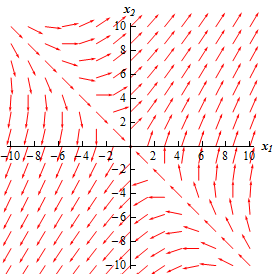Now all we need to do is sketch in some trajectories. To do this all we need to do is remember that the vectors in the sketch above are tangent to the trajectories. Also, the direction of the vectors give the direction of the trajectory as $$t$$ increases so we can show the time dependence of the solution by adding in arrows to the trajectories.

Doing this gives the following sketch.This sketch is called the phase portrait. Usually phase portraits only include the trajectories of the solutions and not any vectors. All of our phase portraits form this point on will only include the trajectories.

In this case it looks like most of the solutions will start away from the equilibrium solution then as $$t$$ starts to increase they move in towards the equilibrium solution and then eventually start moving away from the equilibrium solution again.

There seem to be four solutions that have slightly different behaviors. It looks like two of the solutions will start at (or near at least) the equilibrium solution and then move straight away from it while two other solutions start away from the equilibrium solution and then move straight in towards the equilibrium solution.

In these kinds of cases we call the equilibrium point a saddle point and we call the equilibrium point in this case unstable since all but two of the solutions are moving away from it as $$t$$ increases.

As we noted earlier this is not generally the way that we will sketch trajectories. All we really need to get the trajectories are the eigenvalues and eigenvectors of the matrix $$A$$. We will see how to do this over the next couple of sections as we solve the systems.

Here are a few more phase portraits so you can see some more possible examples. We’ll actually be generating several of these throughout the course of the next couple of sections.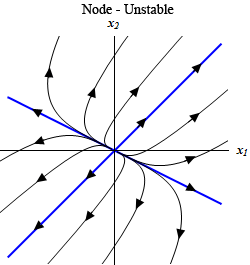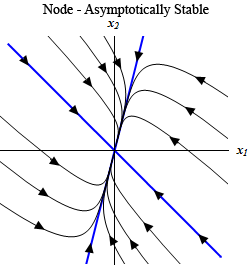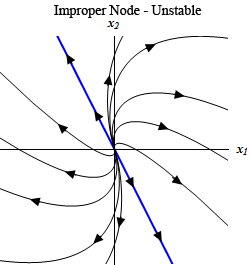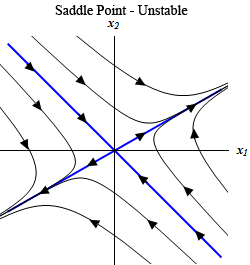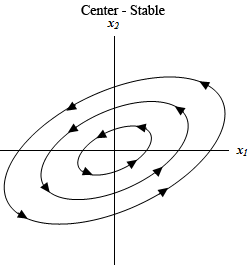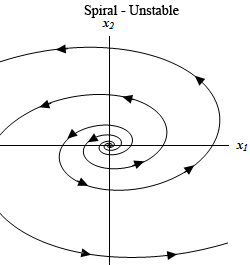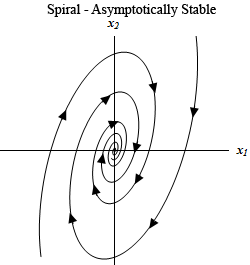Not all possible phase portraits have been shown here. These are here to show you some of the possibilities. Make sure to notice that several kinds can be either asymptotically stable or unstable depending upon the direction of the arrows.

Notice the difference between stable and asymptotically stable. In an asymptotically stable node or spiral all the trajectories will move in towards the equilibrium point as t increases, whereas a center (which is always stable) trajectory will just move around the equilibrium point but never actually move in towards it.